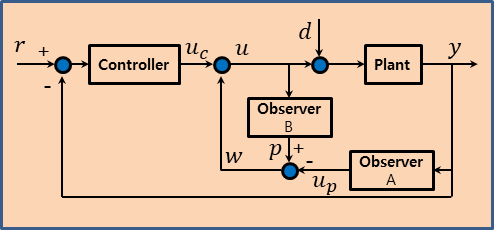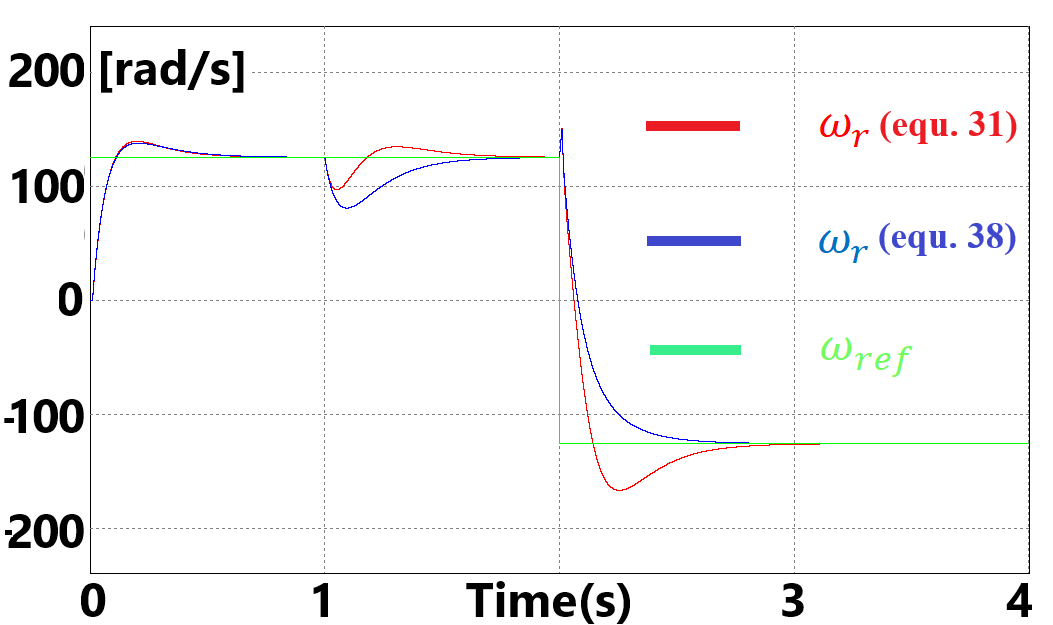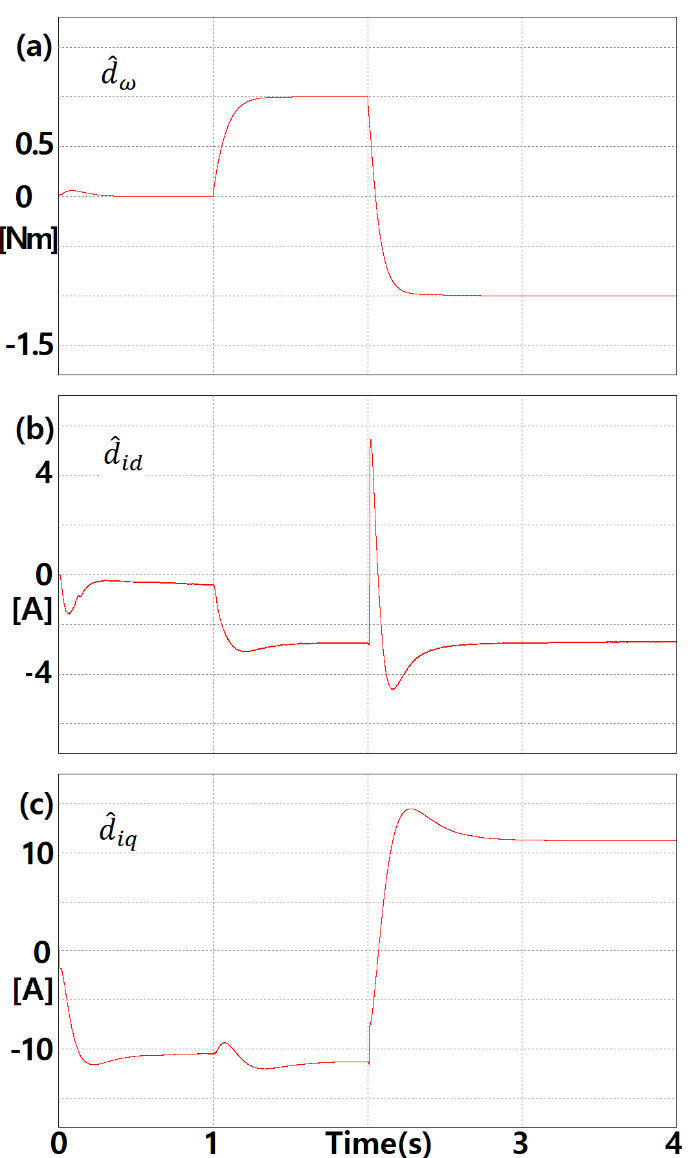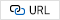Mobile QR Code1. (Department of Aviation Maintenance Engineering, Jungwon University / Chungbuk, Korea waterjiliar@jwu.ac.kr)
2. (Department of Computer Engineering, Jungwon University / Chungbuk, Korea swlee@jwu.ac.kr)

Interior permanent magnet synchronous motor, IPMSM, Disturbance, Disturbance observer, Proportional integral

## 1. Introduction

Electric motors have been widely used in various industrial fields and are now becoming a core part of electric vehicles. They are gradually replacing internal combustion engines. The advantage of using an electric motor is the convenience of their operation and use [1-12]. Two feedback systems are configured to control an interior permanent magnet synchronous motor (IPMSM) [4-12]. One is a control system that controls the position or speed of the motor’s rotation shaft as an output state, and the other is a control system that controls the torque by controlling the current flowing in the stator as an output state. In order to obtain better performance, the mathematical model of the motor must be accurate for precise speed control of the rotating shaft of the motor, and the effect of disturbance acting on the rotating shaft must be considered.

The disturbance estimated from a disturbance observer can be applied to the speed control using a nominal model [1-3]. In this paper, we compensated for the uncertainty of the system model and evaluated the results. An IPMSM can be designed so that disturbance and system uncertainty are removed and control is possible.

## 2. Design of Disturbance observer

In order to obtain precise control performance, a disturbance observer including the disturbance and model error of the mechanical system were designed, and a velocity controller was appropriately configured. Then, the output of the speed controller was set as control reference for a d-axis current controller and a q-axis current controller using the torque formula of the motor. In order to follow a set current control reference, the disturbance observer was designed so that the q-axis current is a disturbance in the d-axis current control, and the d-axis current is a disturbance in the q-axis current control. We propose a system for compensating for disturbance in each current controller.

In the multiple input/output system, the controller design has a large difference between the mechanical time constant and the electrical time constant, making the controller configuration and design of the observer complex and challenging. The primary system of the mechanical equation and the independent primary system of the electrical system are separated into two parts (the d-axis and q-axis) to design the controller. We propose dividing the disturbance observer and designed it according to the state variables so that it can be easily applied. Fig. 1 shows the structure of the disturbance observer.

A mathematical model of the IPMSM is needed to design a disturbance observer to estimate the load torque acting on the rotating shaft of the motor. It is assumed that the magnetoresistance inside the motor is not saturated, there is no iron loss, and the back electromotive force has a sinusoidal shape. The mathematical model of the motor is as follows.

##### (1)
$\frac{d}{dt}\omega _{r}=\frac{1}{J_{m}}T_{e}-\frac{B_{m}}{J_{m}}\omega _{r}-\frac{1}{J_{m}}T_{l} \\$
##### (2)
$\frac{d}{dt}i_{d}=-\frac{R_{s}}{L_{d}}i_{d}+p\frac{L_{q}}{L_{d}}\omega _{r}i_{q}+\frac{1}{L_{d}}V_{d} \\$
##### (3)
$\frac{d}{dt}i_{q}=-\frac{R_{s}}{L_{q}}i_{q}-\frac{pL_{d}}{L_{q}}\omega _{r}i_{d}-\frac{p\varphi _{f}}{L_{q}}\omega _{r}+\frac{1}{L_{q}}V_{q} \\$
##### (4)
$T_{e}=\frac{3}{2}p\left(\varphi _{f}i_{q}+\left(L_{d}-L_{q}\right)i_{d}i_{q}\right)$

$\mathrm{V}_{\mathrm{d}}$ and $\mathrm{V}_{\mathrm{q}}$ are input voltages of the d-axis and q-axis $\mathrm{i}_{\mathrm{d}}$ and $\mathrm{i}_{\mathrm{q}}$ are currents of the d-axis and q-axis, respectively. $\omega _{\mathrm{r}}$ is the mechanical velocity of the rotor, $\mathrm{T}_{\mathrm{e}}$ is the torque caused by electromagnetic, $\mathrm{T}_{\mathrm{l}}$ is the load torque, $\mathrm{R}_{\mathrm{s}}$ is the phase resistance of the stator, and $\mathrm{L}_{\mathrm{d}}$ and $\mathrm{L}_{\mathrm{q}}$ are the inductances of the d-axis and q-axis, respectively. $\varphi _{\mathrm{f}}$ is the flux of the permanent magnet of the rotor, $\mathrm{p}$ is the pole pair of the permanent magnet, $\mathrm{J}_{\mathrm{m}}$ is the moment of inertia of the rotor of the motor, and $\mathrm{B}_{\mathrm{m}}$ is the viscous friction coefficient of the motor rotor.

The IPMSM system considers the load fluctuation of the mechanical system of the rotating shaft of the motor in Eq. (1) as a disturbance and considers the disturbance in the electrical equations (Eqs. (2) and (3)).

##### (5)
$d_{\omega }=T_{l} \\$
##### (6)
$d_{id}=-pL_{q}\omega _{r}i_{q} \\$
##### (7)
$d_{iq}=pL_{d}\omega _{r}i_{d}+p\varphi _{f}\omega _{r}$

To control the torque of the motor, the q-axis current is mainly used, and the d-axis current is controlled to a constant value. We assuming that the d-axis current is controlled by the measurement constant current value C. The Laplace transform of Eq. (4) in Eqs. (1) and (3) is as follows.

##### (8)
$\omega _{r}\left(s\right)=\frac{1}{J_{m}s+B_{m}}T_{e}\left(s\right)-\frac{1}{J_{m}s+B_{m}}d_{\omega }\left(s\right) \\$
##### (9)
$i_{q}\left(s\right)=\frac{1}{L_{q}s+R_{s}}V_{q}-\frac{1}{L_{q}s+R_{s}}d_{iq}\left(s\right) \\$
##### (10)
$T_{e}=\frac{3}{2}p\left(\varphi _{f}+C\left(L_{d}-L_{q}\right)\right)i_{q}\left(s\right)=Ai_{q}\left(s\right)$

We substituted Eq. (9) into Eq. (10) and rearranged it. Then, we substituted Eq. (10) into Eq. (8) to obtain Eq. (11).

##### (11)
$\omega _{r}\left(s\right)=\frac{A}{\left(J_{m}s+B_{m}\right)\left(L_{q}s+R_{s}\right)}\left(V_{q}\left(s\right)-d_{iq}\left(s\right)\right)-\frac{1}{J_{m}s+B_{m}}d_{\omega }\left(s\right)$

The characteristic equation of the transfer function with the angular velocity of the rotating shaft of the motor as the output and the q-axis input voltage as the input is the following.

##### (12)
$\frac{\omega _{r}\left(s\right)}{V_{q}\left(s\right)}=\frac{A}{\left(J_{m}s+B_{m}\right)\left(L_{q}s+R_{s}\right)} \\$
##### (13)
$\frac{\omega _{r}\left(s\right)}{d_{iq}\left(s\right)}=-\frac{A}{\left(J_{m}s+B_{m}\right)\left(L_{q}s+R_{s}\right)} \\$
##### (14)
$\frac{\omega _{r}\left(s\right)}{d_{\omega }\left(s\right)}=-\frac{1}{J_{m}s+B_{m}}$

In this transfer function, we need to design a disturbance observer for Eqs. (13) and (14). Based on Eq. (12), the design of the controller and the observer is a system with a second-order differential and has a complex design process. In order to simplify the design of the disturbance observer and controller, it is advantageous to separate the current system and the mechanical system and to design the system as a first-order differential system. It is also advantageous to check the performance of the designed controller and observer. In addition, the mechanical time constant $\tau _{\mathrm{m}}=J_{m}/B_{m}$ and the electrical time constant $\tau _{e}=L_{q}/R_{s}$ are small values and generally have the following characteristics.

##### (15)
$\tau _{\mathrm{m}}\gg ~ \tau _{e}$

Eq. (15) shows that the response of the mechanical system is slower than that of the electrical system, so the two systems were designed separately, and the electrical system observation and control cycle were designed to be more than 10 times faster than the mechanical system observation and control cycle, which makes it more efficient. The nominal system for Eqs. (1)-(3) of the mechanical system and the electrical system is as follows.

##### (16)
$\frac{d}{dt}\omega _{r}=-\frac{\overline{B_{m}}}{\overline{J_{m}}}\omega _{r}+\frac{1}{\overline{J_{m}}}T_{e} \\$
##### (17)
$\frac{d}{dt}i_{d}=-\frac{\overline{R_{s}}}{\overline{L_{d}}}i_{d}+\frac{1}{\overline{L_{d}}}V_{d} \\$
##### (18)
$\frac{d}{dt}i_{q}=-\frac{\overline{R_{s}}}{\overline{L_{q}}}i_{q}+\frac{1}{\overline{L_{q}}}V_{q}$

The designing of an observer for the nominal system of Eq. (16) is as follows.

##### (19)
$\frac{d}{dt}p_{\omega }=-a_{\omega }p_{\omega }+a_{\omega }u_{\omega } \\$
##### (20)
$\frac{d}{dt}q_{\omega }=-a_{\omega }q_{\omega }+a_{\omega }y_{\omega } \\$
##### (21)
$\hat{u}_{\omega }=\overline{J_{m}}\left(-a_{\omega }q_{\omega }+a_{\omega }y_{\omega }+\frac{\overline{B_{m}}}{\overline{J_{m}}}q_{\omega }\right) \\$
##### (22)
$\hat{d}_{\omega }=p_{\omega }-\hat{u}_{\omega }$

Eq. (19) is used to estimate the output $p_{\omega }$ with respect to the control input under the assumption that the mechanical system has no disturbance. When the transfer function of Eq. (16) is $G_{\omega }$, Eq. (20) is $G_{\omega }^{-1}$ and is used to estimate Eq. (21), which includes disturbance $d_{\omega }$ in the control input. $p_{\omega }$ and $q_{\omega }$ are the state variables of the filter, and $a_{\omega }$ is the gain of the filter. In Eq. (22), the disturbance $\hat{d}_{\omega }$ is estimated by calculating the estimated state $\hat{u}_{\omega }$ of Eq. (21) on the estimated state $p_{\omega }$ of Eq. (19).

The following is the design of the observer for the nominal system of Eq. (17).

##### (23)
$\frac{d}{dt}p_{id}=-a_{id}p_{id}+a_{id}u_{id} \\$
##### (24)
$\frac{d}{dt}q_{id}=-a_{id}q_{id}+a_{id}y_{id} \\$
##### (25)
$\hat{u}_{id}=\overline{L_{d}}\left(-a_{id}q_{id}+a_{id}y_{id}+\frac{\overline{R_{s}}}{\overline{L_{d}}}q_{id}\right) \\$
##### (26)
$\hat{d}_{id}=p_{id}-\hat{u}_{id}$

Eq. (23) is used to estimate the output $p_{id}$ for the control input under the assumption that there is no disturbance $d_{id}$ in the current equation on the d axis. When the transfer function of Eq. (17) is $G_{id}$, Eq. (24) is used to estimate $G_{id}^{-1}$, and Eq. (25) includes disturbance $d_{id}$ in the control input. $p_{id}$ and $q_{id}$ are the state variables of the filter, and $a_{id}$ is the gain of the filter. In Eq. (26), the disturbance $\hat{d}_{id}$ is estimated by calculating the estimated state $\hat{u}_{id}$ of Eq. (25) on the estimated state $p_{id}$ of Eq. (23). Finally, Eq. (18) is the design of the observer for the nominal system.

##### (27)
$\frac{d}{dt}p_{iq}=-a_{iq}p_{iq}+a_{iq}u_{iq} \\$
##### (28)
$\frac{d}{dt}q_{iq}=-a_{iq}q_{iq}+a_{iq}y_{iq} \\$
##### (29)
$\hat{u}_{iq}=\overline{L_{q}}\left(-a_{iq}q_{iq}+a_{iq}y_{iq}+\frac{\overline{R_{s}}}{\overline{L_{q}}}q_{iq}\right) \\$
##### (30)
$\hat{d}_{iq}=p_{iq}-\hat{u}_{iq}$

Eq. (27) is used for estimating the output $p_{iq}$ for the control input under the assumption that there is no disturbance $d_{iq}$ in the current equation on the q axis. When the transfer function of Eq. (18) is $G_{iq}$, Eq. (28) is used to estimate $G_{iq}^{-1}$, and Eq. (29), includes disturbance $d_{iq}$ in the control input. $p_{iq}$ and $q_{iq}$ are the state variables of the filter, and $a_{iq}$ is the gain of the filter. In Eq. (30), the disturbance $\hat{d}_{iq}$ is estimated by calculating the estimated state $\hat{u}_{iq}$ of Eq. (29) on the estimated state $p_{iq}$ of Eq. (27). The estimated disturbance has a structure in which the disturbance is removed by setting it as an input of the plant in addition to the output of the system controller.

##### Fig. 1. The structure of the disturbance observer.## 3. Speed Control

In general, a proportional integral differential (PID) controller is widely used for the position or speed of the rotor of a motor. A PI controller is mainly used in the motor system because a proportional controller improves the steady-state error, and the transient state can achieve a sufficiently good response performance with only the proportional controller. However, in order to improve the response performance of speed control, the disturbance observer was designed in section 2. In order to determine the effect of compensating for the estimated disturbance on the control performance, a velocity controller was designed with only a PI controller and a velocity controller by compensating for the disturbance to the PI controller. The speed controller of the rotor shaft is as follows.

##### (31)
$T_{u}=\overline{J_{m}}\left(k_{\omega p}e_{\omega }+k_{\omega i}\int e_{\omega }dt\right)+\hat{d}_{\omega } \\$
##### (32)
$e_{\omega }=\omega _{ref}-\omega _{r}$

Eq. (31) becomes the target torque value for speed tracking. $k_{\omega p}$ and $k_{\omega i}$ are the gains of the controller, and $\omega _{ref}$ is the target angular velocity. We assumed that the d-axis current is controlled by a constant current value C. Using Eq. (31) in Eq. (4), the q-axis reference current was set as follows.

##### (33)
$i_{q,ref}=T_{u}/\left(\frac{3}{2}p\left(\varphi _{f}+\left(L_{d}-L_{q}\right)C\right)\right)$

Using Eq. (33) as the q-axis current reference, the current controller is designed as follows:

##### (34)
$V_{q}=\overline{L_{q}}\left(k_{iq,p}e_{iq}+k_{iq,i}\int e_{iq}dt\right)+\hat{d}_{iq} \\$
##### (35)
$e_{iq}=i_{q,ref}-i_{q}$

where $k_{iq,p}$ and $k_{iq,i}$ are the gains of the q-axis current controller. The d-axis current controller is as follows:

##### (36)
$V_{d}=\overline{L_{d}}\left(k_{id,p}e_{id}+k_{id,i}\int e_{id}dt\right)+\hat{d}_{id} \\$
##### (37)
$e_{id}=C-i_{d}$

where $k_{id,p}$ and $k_{id,i}$ are the gains of the d-axis current controller. The designed controller in Eq. (31) includes the estimated $\hat{d}_{\omega }$ for the load, and Eqs. (34) and (36) include the estimated disturbances of $\hat{d}_{iq}$ and $\hat{d}_{id}$, respectively. The configuration of a general PI speed controller without disturbance compensation is as follows. The designed control inputs of Eqs. (38), (41) and (43) were designed with a general PI controller and control without disturbance estimation.

##### (38)
$T_{PI}=\overline{J_{m}}\left(k_{\omega p}e_{\omega }+k_{\omega i}\int e_{\omega }dt\right) \\$
##### (39)
$e_{\omega }=\omega _{ref}-\omega _{r} \\$
##### (40)
$i_{q,ref,PI}=T_{PI}/\left(\frac{3}{2}p\left(\varphi _{f}+\left(L_{d}-L_{q}\right)C\right)\right) \\$
##### (41)
$V_{q,PI}=\overline{L_{q}}\left(k_{iq,p}e_{iq}+k_{iq,i}\int e_{iq}dt\right)+\hat{d}_{iq} \\$
##### (42)
$e_{iq}=i_{q,ref,PI}-i_{q} \\$
##### (43)
$V_{d,PI}=\overline{L_{d}}\left(k_{id,p}e_{id}+k_{id,i}\int e_{id}dt\right) \\$
##### (44)
$e_{id}=C-i_{d}$

## 4. Simulation

PSIM was used as a simulation program. The model of the IPMSM uses the model provided in PSIM and the motor parameters in Table 1. The motor parameters are the nominal values.

In a virtual experiment, to determine the effect of compensating for the estimated disturbance on the control performance, the result of speed control with only the PI controller and the result of speed control with the PI controller compensating for the disturbance were compared. When the PI controller with disturbance compensation was designed, the control input used Eqs. (31), (34) and (36). When only the PI controller was designed, the control input utilized Eqs. (38), (41) and (43).

The operation method of the IPMSM motor was started with a reference angular velocity of 125.6 [rad/s] under no load. A constant load of 1 [Nm] was applied at a time of 1 second. Then, it was rotated at an angular velocity of -125.6 [rad/s] at 2 seconds.

The angular velocity shown in red in Fig. 2 is the result of control by Eq. (31) including the disturbance estimated by Eq. (22). The angular velocity displayed in blue is the angular velocity of the motor rotation shaft as a result of controlling the control input using Eq. (38). In the initial startup, all of the controllers show similar performance. As a result of using the control input of Eq. (31), approximately 11.0 [%] overshoot occurred, and as a result of using the control input of Eq. (38), an overshoot of 9.9 [%] occurred. The controllers had similar maneuverability.

However, when a constant load of 1 [Nm] was applied at 1 second, an undershoot of 22.3 [%] occurred as a result of using the control input of Eq. (31). As a result of using the control input of Eq. (38), an undershoot of 35.5 [%] occurred.

As a result of estimating the disturbance and including it in the controller, the undershoot of about 13.2 [%] was improved compared to the controller without compensated disturbance, and it can be judged that the influence of fluctuations in the disturbance acting on the rotating shaft of the motor is reduced. When controlling the motor to rotate at a reverse speed of 125.6 [rad/s] at 2 seconds, the control input of Eq. (31), which compensates for disturbance, advances the rise time by about 0.113 seconds.

The result of disturbance estimated by the designed disturbance observer is shown in Fig. 3. Fig. 3(a) shows the result of estimating the constant load acting on the rotating shaft of the motor with Eqs. (5) and (22). The steady-state estimation error for a constant load of 1 [Nm] acting at 1 second was estimated to be within 0.1 [%]. Fig. 3(b) shows the result of estimating the term of nonlinearity included in the d-axis current of Eq. (6) with Eq. (26). Fig. 3(c) shows the result of estimating the term of the nonlinearity included in the q-axis current of Eq. (7) with Eq. (30).

##### Fig. 2. The speed control result of reference speeds +-125.6 [rad/s].##### Fig. 3. Disturbance estimation result.##### Table 1. IPMSM parameters.
 Parameters Values Motor Rated Power 3-phase 1 hp Motor Rated Speed 1200 RPM $\mathrm{P}$ (Pole Number) 4 $\mathrm{R}_{\mathrm{s}}$ (Stator Resistance) 0.048 Ω $\mathrm{L}_{\mathrm{d}}$ (D-axis Inductance) 0.42 mH $\mathrm{L}_{\mathrm{q}}$ (Q-axis Inductance) 1.2 mH $\mathrm{J}_{\mathrm{m}}$ (Moment of Inertia) 0.0008 Kg m2 $\mathrm{B}_{\mathrm{m}}$ (Friction Coefficient) 0.001 Nm/rad/s $\varphi _{\mathrm{f}}$ (Magnetic Flux Constant) 0.04135 volt/rad/s
##### Table 2. Speed control result.
 PI controller with D.O.B PI controller without D.O.B Overshoot after the beginning 11.0 [%] 9.0 [%] Undershoot after 1.0s 22.3 [%] 35.5 [%] Rise time after 2.0s 0.109 [s] 0.222 [s]

## 5. Conclusion

A PI controller is commonly used in controlling the speed or current of a motor and overcomes the disturbance slowly. To improve this, we estimated the disturbance and compensated it with a PI controller so that the sloshing becomes smaller and the disturbance is quickly overcome. In order to improve the control performance of the speed of the IPMSM, a disturbance observer was designed to reduce the effect of disturbance. With the same control gains, the control result including the disturbance estimated from the designed observer in the controller improved the undershoot by about 13.2 [%] or more compared to the result using only the pure PI controller. In the future, it will be necessary to conduct research on a controller that is robust against the error of the parameters of the nominal system and can accurately estimate the disturbance even if the speed and current state contain noise.

### ACKNOWLEDGMENTS

This research was supported by the Jungwon University Research Grant (No. 2020-026).

### REFERENCES

1
K. Ohnishi, “A new servo method in mechatronics,” Trans. of Japanese Society of Electrical Engineers, vol. 107-D, pp. 83-86, 1987.2
J. Back and H. Shim, “Adding robustness to nominal output feedback controllers for uncertain nonlinear systems: A nonlinear version of disturbance observer,” Automatica, vol. 44, no. 10, pp. 2528-2537, 2008.3
J. Back and H, Shim, “An inner-loop controller guaranteeing robust transient performance for uncertain MIMO nonlinear systems,” IEEE Trans. on Automatic Control, vol. 54, no. 7, pp. 1601-1607, 2009.4
J. Kim, “A robust sensorless speed control of sensorless BLDC motor,” J. of the Korea Institute of Electronic Communication Sciences, vol. 3, no. 4, pp. 266-275, 2008.5
H. Lee, W. Cho, and K. Lee, “Improved switching method for sensorless BLDC motor drive,” J. of the Korea Institute of Electronic Communication Sciences, vol. 5, no. 2, pp. 164-170, 2010.6
Y-H. Jeon and M-H. Cho, “A Speed Control of BLDC Motor using Adaptive Back stepping Technique,” J. of the Korea Institute of Electronic Communication Sciences, vol. 9, no. 8, pp. 899-905, 2014.7
J. Zhou and Y. Wang, “Adaptive backstepping speed controller design for a permanent magnet synchronous motor,” Electric Power Applications IEE Proc. vol. 149, no. 2, pp. 165-172, 2002.8
M. Ouassaid, M. Cherkaoui, and Y. Zidani, “A Nonlinear Speed Control for a PM Synchronous Motor Using an Adaptive Back -stepping Control Approach,” IEEE Int. Conf. on Industrial Technology (ICIT), Hammamet, Tunisia, vol. 3, pp. 1287-1292, 2004.9
S. Rebouh, A. Kaddouri, R. Abdessemed, and A. Haddoun, “Adaptive Back stepping speed Control for a Permanent Magnet Synchronous Motor,” Management and Service Science (MASS) 2011 Int. Conf., Wuhan, China, pp. 1-4, 2011.10
L. Yuan, H. Feng-you, and W. Feng “Nominal Model-Based Control for Permanent Magnet Synchronous Motor,” 2009 Int. Conf. on Intelligent Human-Machine Systems and Cybernetics, Hangzhou, China, vol. 2, pp. 343-346, 2009.11
S. Back, “A Study on the Design and Implementation of 2-phase BLDC Fan Motor with 1-horsepower Class for Air Conditioning,” J. of the Korea Institute of Electronic Communication Sciences, vol. 13, no. 4, Aug., pp. 760, 2018.12
H. Kwon, “Knee Rehabilitation System through EMG Signal analysis and BLDC Motor Control,” J. of the Korea Institute of Electronic Communication Sciences, vol. 14, no.5, Oct., pp. 1009-1018, 2019.## Author

Yongho Jeon received his B.S. and M.S. degrees in Control and Instrumentation Engineering from Kwangwoon University, Rep. of Korea, in 1996 and 1998, respectively and his Ph.D. in Information and Control Engineering from the University of Kwangwoon in 2008. Since 2013, he has been an Associate Professor with department of Aviation Maintenance at the Jungwon University, Chungbuk, South Korea. His research interests include variable speed system, intelligent robot system and control system.

Shinwon Lee received his B.S. and M.S. degrees in Computational Statistics from Jeonbuk National University, Rep. of Korea, in 1990 and 1992, respectively and her Ph.D. in Computer Science and Engineering from Jeonbuk National University in 2005. She worked as an assistant professor in the Department of Computer Information at Jeonbuk Science College from 1995 to 2004. Since 2009, she has been an Associate Professor with department of Computer Engineering at the Jungwon University, Chungbuk, South Korea. Her research interests include control system, ICT, machine learning, and artificial Intelligence.